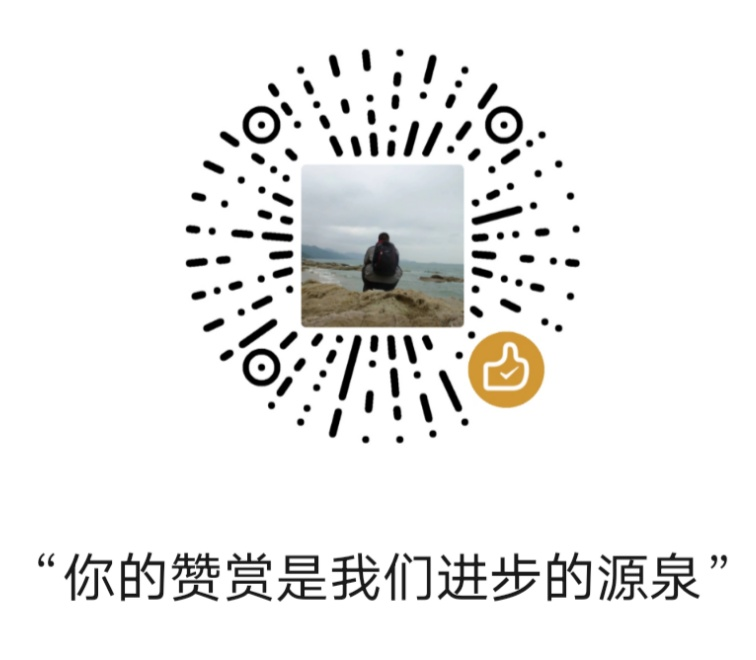# 第三讲 JavaScript程序构成

JavaScript脚本语言的基本构成是由控制语句、函数、对象、方法、属性等,来实现编程的。

、if条件语句

if（表述式）

......
else

.....

if -else 语句是JavaScript中最基本的控制语句，通过它可以改变语句的执行顺序。

if语句的嵌套
if（布尔值）语句１；
else（布尔值）语句２；
else if（布尔值）语句３；
……
else 语句４；

、For循环语句

for（初始化；条件；增量）

、while循环

while（条件）

For与while语句

、break和continue语句

、JavaScript函数定义

Function 函数名 （参数,变元）{

Return 表达式;
}

、函数中的形式参数：

Function function_Name(exp1,exp2,exp3,exp4)
Number =function _Name . arguments .length;
if (Number>1）
document.wrile(exp2);
if (Number>2)
document.write(exp3);
if(Number>3)
document.write(exp4);
...

、基本概念

JavaScript是基于对象(object-based)的语言。这与Java不同,Java是面向对象的语言。而基于对象的基本特征，就是采用事件驱动(event-driven)。它是在用形界面的环境下，使得一切输入变化简单化。通常鼠标或热键的动作我们称之为事件（Event），而由鼠标或热键引发的一连串程序的动作，称之为事件驱动（Event Driver）。而对事件进行处理程序或函数，我们称之为事件处理程序（Event Handler）。

、事件处理程序

Function 事件处理名（参数表）{

……
}

、事件驱动

JavaScript事件驱动中的事件是通过鼠标或热键的动作引发的。它主要有以下几个事件：

（１）单击事件onClick

button（按钮对象）
checkbox（复选框）或（检查列表框）
reset buttons（重要按钮）
submit buttons（提交按钮）

<Form>
<Input type="button" Value=“ ” onClick="change()">
</Form>

<Input type="button" value=" " onclick=alert("这是一个例子"）;

（２）onChange改变事件

<Form>
<Input type="text" name="Test" value="Test" onCharge="check('this.test)">
</Form>

（３）选中事件onSelect

（４）获得焦点事件onFocus

（５）失去焦点onBlur

test3_1.htm

<HTML>
<script Language="JavaScript">
<!--
}
}
//-->
</Script>
<a href="test.htm">调用</a>
</BODY>
</HTML>

test3_2.htm

<html>
<script language="JavaScript"><!--
// -->
function makeArray(n){
this.length=n
return this
}
function hexfromdec(num) {
hex=new makeArray(1);
var hexstring="";
var shifthex=16;
var temp1=num;
for(x=1; x>=0; x--) {
hex[x]=Math.round(temp1/shifthex - .5);
hex[x-1]=temp1 - hex[x] * shifthex;
temp1=hex[x-1];
shifthex /= 16;
}
for (x=1; x>=0; x--) { hexstring+=getletter(hex[x]); }
return (hexstring);
}
function getletter(num) {
if (num < 10) { return num; }
else {
if (num == 10) { return "A" }
if (num == 11) { return "B" }
if (num == 12) { return "C" }
if (num == 13) { return "D" }
if (num == 14) { return "E" }
if (num == 15) { return "F" }
}
}
function rainbow(text){
var color_d1;
var allstring="";
for(i=0;i<text.length;i=i+2){
color_d1=255*Math.sin(i/(text.length/3));
color_h1=hexfromdec(color_d1);
allstring+="<FONT COLOR="+color_h1+"ff"+color_h1+">"+text.substring(i,i+2)+"</FONT>";
}
return allstring;
}
function sizefont(text){
var color_d1;
var allstring="";
var flag=0;
for(i=0,j=0;i<text.length;i=i+1){
if (flag==0) {
j++;
if (j>=7) {
flag=1;}}
if (flag==1) {
j=j-1;
if (j<=0) {
flag=0; }}
allstring+="<FONT SIZE="+ j + ">" + text.substring(i,i+1) + "</FONT>";
}
return allstring;
}
document.write("<font size=8><CENTER>")
document.write("<BR><BR>")
document.write( sizefont("这是一个获取WEB浏览器的程序"))
document.write("</CENTER></font>")
document.write("浏览器名称: "+navigator.appName+"<br>");
document.write("版本号: "+navigator.appVersion+"<br>");
document.write("代码名字: "+navigator.appCodeName+"<br>");
document.write("用户代理标识: "+navigator.userAgent);
</script>
<body>
</body>
</html>